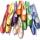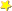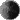Forum:Precalculus Function Question Help!
By iv_loves_pointeOn Sun Nov 24, 2013 09:37 PM

I've been trying to figure this out for two days and I can't. Any assistance will be greatly appreciated. This question is due tomorrow. Please show all work. Thank you very much!

Express the area A of a an equilateral triangle as a function of perimeter P.

### 1 Replies to Precalculus Function Question Help!

re: Precalculus Function Question Help!
By Panda_BearOn Sun Nov 24, 2013 09:50 PM
area of a triangle = bh/2

equilateral triangle means that all 3 sides are equal. peremtrer = S1+s2+s3.

we know that s1,s2,s3 = b

calculate the height

h= inverse cos (b/2)/b

b= 1/3p

A(p)= (1/3p*h)/2